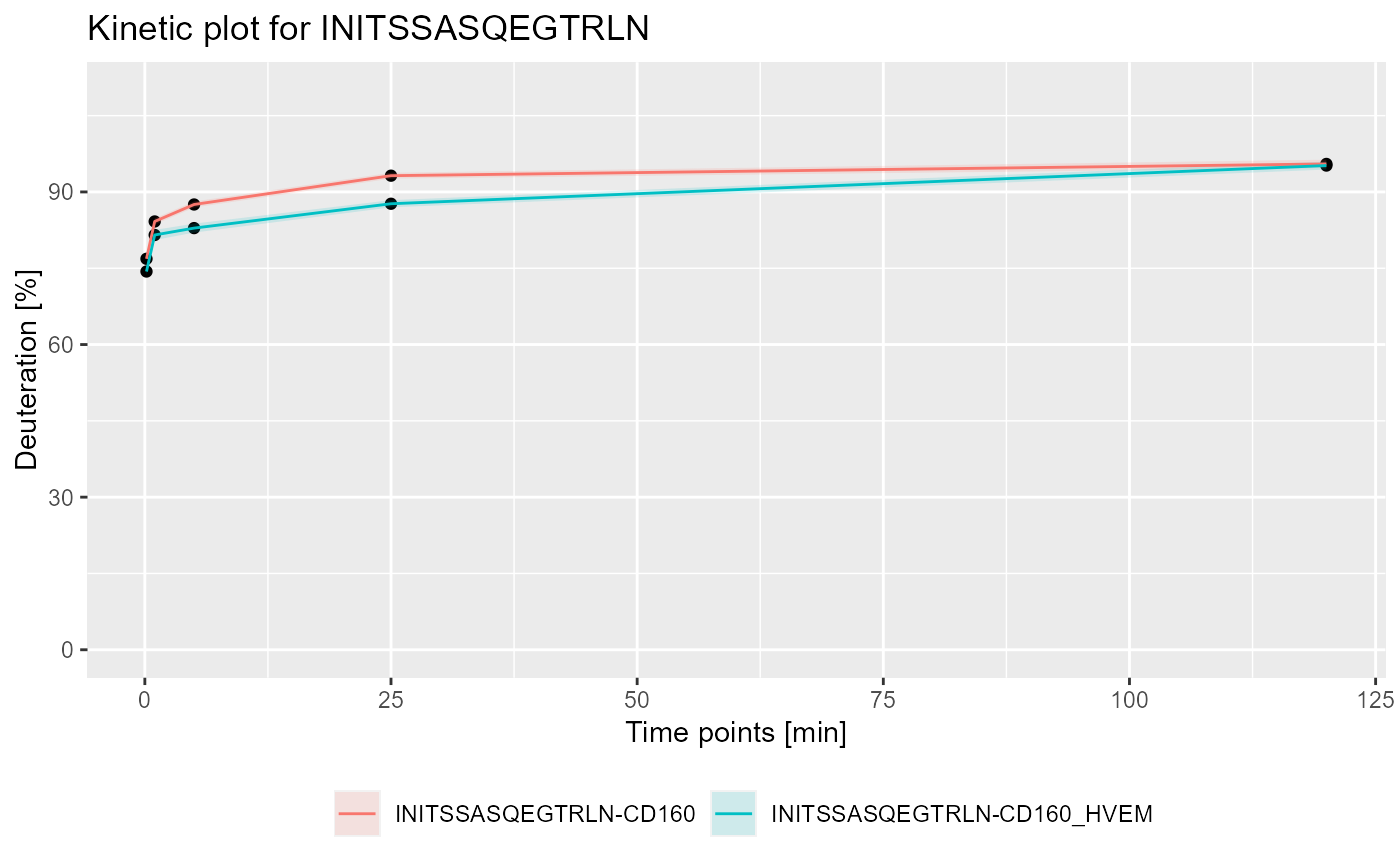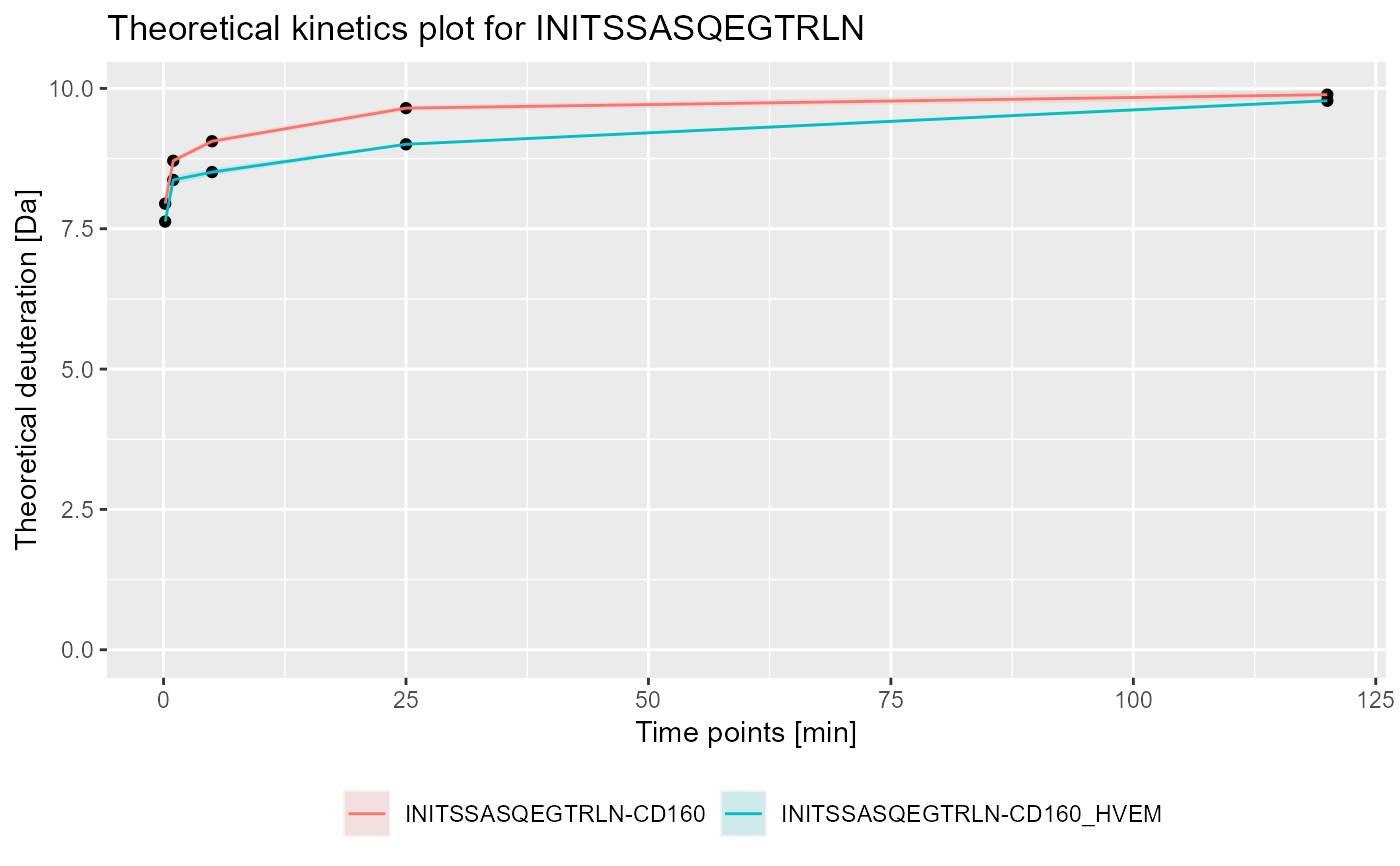Calculate kinetics of the hydrogen-deuteration exchange for given peptide.

calculate_kinetics(
dat,
protein = dat[["Protein"]],
sequence,
state,
start,
end,
time_in,
time_out,
deut_part = 1
)

## Arguments

dat dat data read by read_hdx protein value for chosen peptide sequence of the peptide for which the kinetics is calculated state of given sequence end of given sequence end of given sequence time in for experimental calculations time out for experimental calculations percentage of deuterium the protein was exposed to, value in range [0, 1]

## Value

data frame with deuteration calculated for all the data points between time_in and time_out. The chosen time point for which deuteration in all four variants is calculated is available in column time_chosen. The rest of the returned structure is equivalent to structure returned by calculate_state_deuteration.

## Details

The function calculates deuteration data for all available data points for given peptide. All four variants (relative & theoretical combinations) of deuteration computations are supported. Manual correction of percentage of deuterium the protein was exposed to during the exchange in theoretical calculations is provided. To visualize obtained data we recommend using plot_kinetics function. The first version doesn't support filled Modification and Fragment columns.

read_hdx calculate_state_deuteration plot_kinetics

## Examples

# load example data

# calculate data for sequence INITSSASQEGTRLN in state CD160
(kin1 <- calculate_kinetics(dat,
protein = "db_CD160",
sequence = "INITSSASQEGTRLN",
state = "CD160",
start = 1,
end = 15,
time_in = 0.001,
time_out = 1440))
#> # A tibble: 5 x 15
#>   Protein Sequence Start   End State time_chosen frac_exch_state
#>   <chr>   <chr>    <int> <int> <chr>       <dbl>           <dbl>
#> 1 db_CD1~ INITSSA~     1    15 CD160       0.167            76.9
#> 2 db_CD1~ INITSSA~     1    15 CD160       1                84.2
#> 3 db_CD1~ INITSSA~     1    15 CD160       5                87.5
#> 4 db_CD1~ INITSSA~     1    15 CD160      25                93.2
#> 5 db_CD1~ INITSSA~     1    15 CD160     120                95.5
#> # ... with 8 more variables: err_frac_exch_state <dbl>,
#> #   abs_frac_exch_state <dbl>, err_abs_frac_exch_state <dbl>,
#> #   avg_theo_in_time <dbl>, err_avg_theo_in_time <dbl>,
#> #   abs_avg_theo_in_time <dbl>, err_abs_avg_theo_in_time <dbl>,
#> #   Med_Sequence <dbl>
# calculate data for sequence INITSSASQEGTRLN in state CD160_HVEM
(kin2 <- calculate_kinetics(dat,
protein = "db_CD160",
sequence = "INITSSASQEGTRLN",
state = "CD160_HVEM",
start = 1,
end = 15,
time_in = 0.001,
time_out = 1440))
#> # A tibble: 5 x 15
#>   Protein Sequence Start   End State time_chosen frac_exch_state
#>   <chr>   <chr>    <int> <int> <chr>       <dbl>           <dbl>
#> 1 db_CD1~ INITSSA~     1    15 CD16~       0.167            74.4
#> 2 db_CD1~ INITSSA~     1    15 CD16~       1                81.5
#> 3 db_CD1~ INITSSA~     1    15 CD16~       5                82.9
#> 4 db_CD1~ INITSSA~     1    15 CD16~      25                87.7
#> 5 db_CD1~ INITSSA~     1    15 CD16~     120                95.2
#> # ... with 8 more variables: err_frac_exch_state <dbl>,
#> #   abs_frac_exch_state <dbl>, err_abs_frac_exch_state <dbl>,
#> #   avg_theo_in_time <dbl>, err_avg_theo_in_time <dbl>,
#> #   abs_avg_theo_in_time <dbl>, err_abs_avg_theo_in_time <dbl>,
#> #   Med_Sequence <dbl>
library(dplyr)
#>
#> Dołączanie pakietu: 'dplyr'#> Następujące obiekty zostały zakryte z 'package:stats':
#>
#>     filter, lag#> Następujące obiekty zostały zakryte z 'package:base':
#>
#>     intersect, setdiff, setequal, unionlibrary(ggplot2)
#> Warning: pakiet 'ggplot2' został zbudowany w wersji R 4.0.3
# plot example - experimental and relative
bind_rows(kin1, kin2) %>%
plot_kinetics(theoretical = FALSE,
relative = TRUE) +
labs(title = "Kinetic plot for INITSSASQEGTRLN")# plot example - theoretical and absolute
bind_rows(kin1, kin2) %>%
plot_kinetics(theoretical = TRUE,
relative = FALSE) +
labs(title = "Theoretical kinetics plot for INITSSASQEGTRLN")